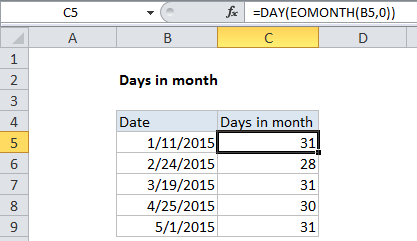## Excel Office

Excel How Tos, Tutorials, Tips & Tricks, Shortcuts

# Display Days in month in Excel

This tutorial shows how to Display Days in month in Excel using example below.

To get the number of days in a given month from a date, you can use a formula based on the EOMONTH and DAY functions.

Worked Example:   Get last weekday in month in Excel

## Formula

`=DAY(EOMONTH(date,0))`## Explanation

In the example shown, the formula in cell B5 is:

`=DAY(EOMONTH(B5,0))`

### How this formula works

The DAY function returns the day component of a date.  The EOMONTH function returns the last day of the month for a given date. So, in this formula EOMONTH first returns a date corresponding to the last day of the month, and then DAY returns the date value for that date.

Worked Example:   Average by month in Excel

By definition, the value returned by DAY is always equal to the number of days in the month, since the date supplied to DAY is always the last day.

Worked Example:   EOMONTH function: Description, Usage, Syntax, Examples and Explanation